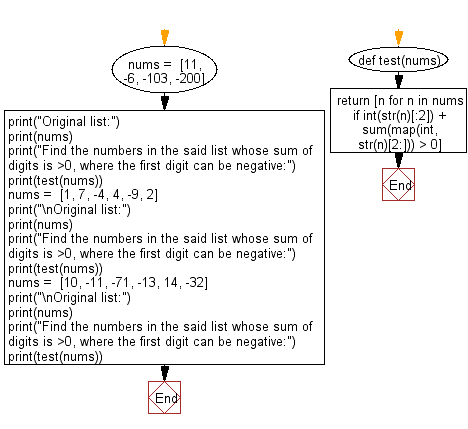﻿ Python: Filter for the numbers in a list whose sum of digits is >0, where the first digit can be negative - w3resource# Python: Filter for the numbers in a list whose sum of digits is >0, where the first digit can be negative

## Python Programming Puzzles: Exercise-47 with Solution

Write a Python program to Filter for the numbers in numbers in a given list whose sum of digits is > 0, where the first digit can be negative.

```Input:
[11, -6, -103, -200]
Output:
[11, -103]

Input:
[1, 7, -4, 4, -9, 2]
Output:
[1, 7, 4, 2]

Input:
[10, -11, -71, -13, 14, -32]
Output:
[10, -13, 14]
```

Sample Solution:

Python Code:

``````#License: https://bit.ly/3oLErEI

def test(nums):
return [n for n in nums if int(str(n)[:2]) + sum(map(int, str(n)[2:])) > 0]
nums =  [11, -6, -103, -200]
print("Original list:")
print(nums)
print("Find the numbers in the said list whose sum of digits is >0, where the first digit can be negative:")
print(test(nums))
nums =  [1, 7, -4, 4, -9, 2]
print("\nOriginal list:")
print(nums)
print("Find the numbers in the said list whose sum of digits is >0, where the first digit can be negative:")
print(test(nums))
nums =  [10, -11, -71, -13, 14, -32]
print("\nOriginal list:")
print(nums)
print("Find the numbers in the said list whose sum of digits is >0, where the first digit can be negative:")
print(test(nums))
``````

Sample Output:

```Original list:
[11, -6, -103, -200]
Find the numbers in the said list whose sum of digits is >0, where the first digit can be negative:
[11, -103]

Original list:
[1, 7, -4, 4, -9, 2]
Find the numbers in the said list whose sum of digits is >0, where the first digit can be negative:
[1, 7, 4, 2]

Original list:
[10, -11, -71, -13, 14, -32]
Find the numbers in the said list whose sum of digits is >0, where the first digit can be negative:
[10, -13, 14]
```

Flowchart:## Visualize Python code execution:

The following tool visualize what the computer is doing step-by-step as it executes the said program:

Python Code Editor :

Have another way to solve this solution? Contribute your code (and comments) through Disqus.

What is the difficulty level of this exercise?

Test your Programming skills with w3resource's quiz.

﻿

## Python: Tips of the Day

Clamps num within the inclusive range specified by the boundary values x and y:

Example:

```def tips_clamp_num(num,x,y):
return max(min(num, max(x, y)), min(x, y))
print(tips_clamp_num(2, 4, 6))
print(tips_clamp_num(1, -1, -6))
```

Output:

```4
-1
```